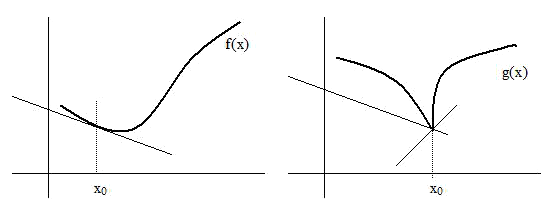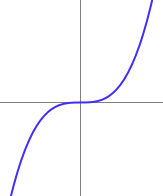# Derivates, Locale extreme, inflected and saddle points

### Basic concepts and principles

The derivative of a function at a point measures the rate of change of the function at that point. We will see in this section this essential concept in physics and many other sciences in which Maths are applied.

Mathematical definition of derivate of a function f, at a point x0 is

$f'(x_{0}) = \lim_{x \to 0} \frac{f(x_{0}+h) - f(x_{0})}{h}$

Equivalently by changing variable h=x-x0

$f'(x_{0})= \lim_{x \to x_{0}}{ \frac{f(x)-f(x_{0}) }{x-x_{0}}}$

if limits does not exists it is said thaf f is not derivable at x0.

Geometrically it is usual to introduce the concept of derivative as the limit of secants rects as follows

The limit of these secants is called tangent rect to the function at the point x0.

Another one approach to derivative concept is that it exists if the graph of the function is soft at that point where we mean by "soft" that is continuous and has not corners and nor jumps at that point.

An important fact easy to show directly from the definition is the following theorem

### Theorem

IF f is derivable at x0, then f is continous at x0
The converse of previous theorem is not true, as we shall see in the following example:Both f and g are continuous at x0, But f is differentiable and g not.

A typical example of continuous but not differentiable function at x = 0 is the function

$f(x)=|x|$

$f'(x) = \left\{\begin{matrix} -1 & x<0\\ 1 & x>0 \end{matrix}\right.$

$f'(0)=lim_{h \to 0}{\frac{f(0+h) - f(0)}{h}} = lim_{h \to 0}{\frac{|h| - |0|}{h}} = \frac{|h|}{h}$

This limit value 1 if h>0 and -1 if h< 0. When this occurs we are talking about rigth limit and left limit, Because they are different at x=0, the limit does not exists at x=0.

### Exthended Theory

f'(x) is also a function, it is possible to define f''(x0) as

$f''(x_{0})=lim_{h\rightarrow 0}{\frac{f'(x_{0} + h) - f'(x_{0})}{h}}$

In those points x where this limit exists, it is said that f is twice differentiable at x.

And similarly it is possible to define f'''(x), f(iv)(x), and so on ...

### Increasing and decreasing intervals of a function

A differentiable function f, is said to be increasing at an interval I= [a, b], if x1, x2 ∈ I with x1< x2 then f(x1) ≤ f(x2).
And, f es decreasing if x1, x2 ∈ I with x1< x2 then f(x1) ≥ f(x2).

Note:sometimes it is typed in the above definition a minor (or less) strict, at these cases it is said that the function is strictly increasing (or decreasing)If f is an increasing function at interval [a, b] its derivate is positive is positive, ie

$f'(x) \geq 0 ,\ \forall x \in [a,b]$

So, this again justifies what we said at the beginning of this section: the derivative of a function measures the rate of change of the function.

### Local extreme and saddle pointsConsider a differentiable function f.

If f has a local minimum at a point x0, the function is decreasing when values of x are some less than x0and increasing for values some greater than x0. Thus derivative is negative in any environment on the left of x0 and derivate is positive for x to the right of x0. More precisely:

### f(x) has a local minimum at x= x0

then

$f'(x) <0 ,\ \forall x \in (x_{0}- \epsilon, x_{0} )$

$f'(x) > 0 ,\ \forall x \in (x_{0}, x_{0} + \epsilon )$

Similarly, if the function has a local maximum at x1 the derivative is positive in an environment to the left of x1 and negative in a right of x1:

### f(x) has a local maximum at x= x1

then

$f'(x) >0 ,\ \forall x \in (x_{1}- \epsilon, x_{1} )$

$f'(x) < 0 ,\ \forall x \in (x_{1}, x_{1} + \epsilon )$

In both cases, maximum and minimum at x0 it is holds

$f'(x_{0}) = f'(x_{1}) = 0$

And converse is truth? NO!!, if f'(x0) = 0, function may be a maximum, minimum, or what is called a saddle point, this last, is the case of the function f(x)=x3 for x=0.Graph of y=x3, x=0 is a saddle point because it is not local maximum and nor local minimum but y'=0

In general, a point where f'(x0) = 0 is called a critical point (which may be a maximum, minimum or a saddle point)

### Convexity, concavity and inflection points

This idea also that can help us to decide if a critical point is a maximum, minimum or sadlle point and is related with the second derivative. Lets suposse that f is a function twice derivable with continuity.

If the second derivative of f is positive at x0, then f is convex in any enviroment arroud x0. On the contrary, if f'' is negative, then function is concave at any interval containing x0.

A point x0 where a function changes from concave to convex or from convex to concave is inflection point, also turning point, inflection point (do not to confuse this concept with the saddle point)Again y=x3, x=0 is aninflection point because the function is convex over the interval (-∞, 0) and concave over (0, ∞)

So, given a function f twice differentiable, and critical point x0 of f (therefore remember f'(x0) = 0 ) it is holds

If $f''(x_{0})<0$ then x0 is a local maximum.

If $f''(x_{0})> 0$ then x0 is a local manimum.

If $f''(x_{0}) = 0$ then x0 could be maximum, minimum or saddle point.

### F, F' and F'' representation and relationships

 See behavior F, F' and F'' at each singular point and each intervalPoints x0 x2 x1 F(x) Minimum Inflection Point Maximum F'(x) Zero Maximum Zero F''(x) Positive value Zero Negative value Interval (-∞, x0] [x0, x2] [x2, x1] [x1, ∞) F(x) Decreasing, Convex Increasing, Convex Increasing, Concave Decreasing, Concave F'(x) Increasing, Negative Increasing, Positive Decreasing, Positive Decreasing, Negative F''(x) Positive Positive Negative Negative

# Was useful? want add anything?

Post here

### Post from other users

Post here